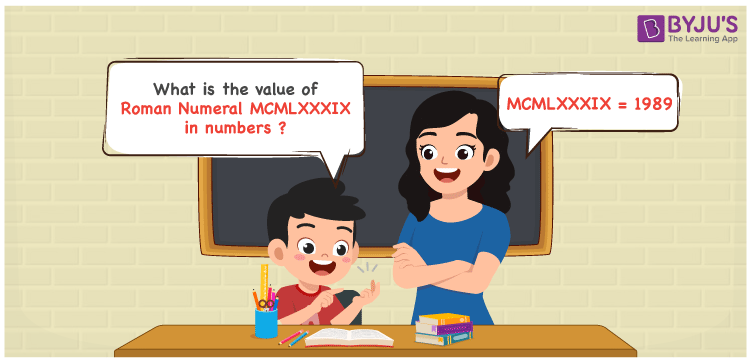Checkout JEE MAINS 2022 Question Paper Analysis : Checkout JEE MAINS 2022 Question Paper Analysis :

# MCMLXXXIX Roman Numerals

MCMLXXXIX Roman Numerals is 1989. We know that Roman Numerals are the system of expressing numbers using the Roman alphabet. Practising the problems using the PDF of Roman Numerals 1 to 1000 on a regular basis helps students to solve complex problems with ease. Learn how to express the numerical value of MCMLXXXIX Roman Numerals using a simple method in this article.

 Number Roman Numeral 1989 MCMLXXXIX

## How to Write MCMLXXXIX Roman Numerals?The following methods can be used to denote the value of MCMLXXXIX Roman Numerals with ease

First Method:

• Break the Roman Numerals into single letters
• MCMLXXXIX = M + (M – C) + L + X + X + X + (X – I)
• Write the numerical value of each letter and add/subtract them
• MCMLXXXIX = 1000 + (1000 – 100) + 50 + 10 + 10 + 10 + (10 – 1) = 1989

Second Method:

In this method, we consider the groups of Roman Numerals for addition or subtraction

• MCMLXXXIX = M + CM + LXXX + IX = 1000 + 900 + 80 + 9 = 1989
• Therefore the numerical value of MCMLXXXIX Roman Numerals is 1989

## Video Lesson on Roman Numerals## Related Articles

Roman Numerals

Roman Numerals 1 to 50

Roman Numerals 1 to 100

Roman Numerals Conversion

L Roman Numerals

Roman Numeral XXXIX

## Frequently Asked Questions on MCMLXXXIX Roman Numerals

### What is MCMLXXXIX Roman Numerals?

MCMLXXXIX Roman Numerals is 1989.

### What is the value of 1900 + 89 in Roman Numerals?

1900 + 89 = 1989. The number 1989 in Roman Numerals is MCMLXXIX. Hence the value of 1900 + 89 in Roman Numerals is MCMLXXXIX.

### What is the difference between 1989 and 1900 in Roman Numerals?

1989 – 1900 = 89. The number 89 in Roman Numerals is LXXXIX. Thus the difference between 1989 and 1900 in Roman Numerals is LXXXIX.